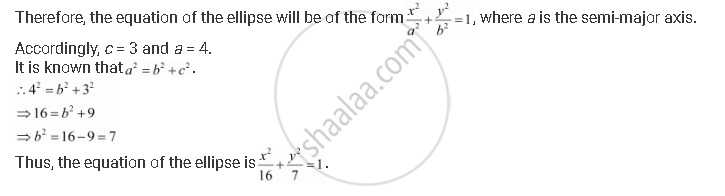CBSE (Arts) Class 11CBSE
Share
Notifications

View all notifications
Books Shortlist
Your shortlist is empty

# Find the Equation for the Ellipse that Satisfies the Given Conditions: Foci (±3, 0), a = 4 - CBSE (Arts) Class 11 - Mathematics

Login
Create free account

Forgot password?
ConceptEllipse Standard Equations of an Ellipse

#### Question

Find the equation for the ellipse that satisfies the given conditions: Foci (±3, 0), a = 4

#### Solution

Foci (±3, 0), a = 4

Since the foci are on the x-axis, the major axis is along the x-axis.Is there an error in this question or solution?

#### APPEARS IN

Solution Find the Equation for the Ellipse that Satisfies the Given Conditions: Foci (±3, 0), a = 4 Concept: Ellipse - Standard Equations of an Ellipse.
S